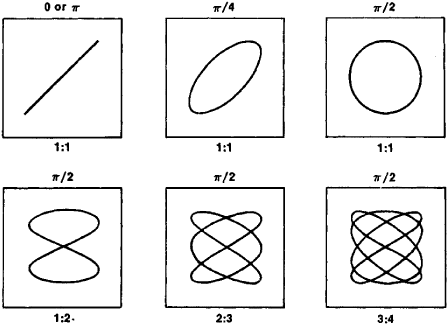Lissajous Figures

Also found in: Dictionary.

Lissajous figures

Plane curves traced by a point which executes two independent harmonic motions in perpendicular directions, the frequencies of the motion being in the ratio of two integers. Such figures are widely used in frequency and phase measurements (see illustration). See Harmonic motion

The cathode-ray oscilloscope furnishes the most important and practical means for the generation of the figures. The x-deflection plates of the tube are supplied with one alternating voltage, and the y-deflection plates with another. If the frequencies are incommensurable, the figure is not a closed curve and, except for very low frequencies, will appear as a patch of light because of the persistence of the screen. On the other hand, if the frequencies are commensurable, the figure is closed and strictly periodic; it is a true Lissajous figure, stationary on the screen and, if the persistence is sufficient, visible continuously as a complete pattern. See Frequency measurement, Oscilloscope, Phase-angle measurement

McGraw-Hill Concise Encyclopedia of Physics. © 2002 by The McGraw-Hill Companies, Inc.
The following article is from The Great Soviet Encyclopedia (1979). It might be outdated or ideologically biased.

Lissajous Figures

the closed paths described by a point that simultaneously performs two harmonic oscillations in two mutually perpendicular directions. They were first studied by the French scientist J. Lissajous (1822–80). The shape of Lissajous figures depends on the ratio of the periods (frequencies), phases, and amplitudes of the two oscillations. In the simplest case (equal periods), the Lissajous figures are ellipses that degenerate into line segments for a phase difference of 0 or π and reduce to a circle when the phase difference is π/2 and the amplitudes areFigure 1. Shape of Lissajous figures for various ratios of periods (1:1, 1:2, and so on) and phase differences

equal (see Figure 1). If the periods of the two oscillations do not coincide exactly, the phase difference and, consequently, the shape of the ellipse change continuously.

Lissajous figures are not observed if the periods differ substantially. However, if the periods are related as integers, the moving point will return to its original position in a period of time equal to the least multiple of the two periods, and a Lissajous figure with a more complex shape is produced.

Lissajous figures may be observed on the screen of a cathoderay oscilloscope. They result from the displacement of a luminous point if alternating voltages that are either equal or multiples of each other are fed to two pairs of deflector plates. The observation of Lissajous figures is a convenient method for studying the ratios of the periods and phases of oscillations, as well as the shape of the oscillations.